[Introduction]  [Signal arithmetic]  [Signals and noise]   [Smoothing]   [Differentiation]  [Peak Sharpening]  [Harmonic analysis]   [Fourier convolution]  [Fourier deconvolution]  [Fourier filter]  [Wavelets]   [Peak area measurement]  [Linear Least Squares]  [Multicomponent Spectroscopy]  [Iterative Curve Fitting]  [Hyperlinear quantitative absorption spectrophotometry] [Appendix and Case Studies]  [Peak Finding and Measurement]  [iPeak]   [iSignal]  [Peak Fitters]   [iFilter]  [iPower]  [List of downloadable software]  [Interactive tools]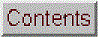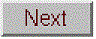## Harmonic analysis and the Fourier Transform

Some signals exhibit periodic components that repeat at fixed intervals throughout the signal, like a sine wave. It is often useful to describe the amplitude and frequency of such periodic components exactly. Actually, it is possible to analyze any arbitrary set of data into periodic components, whether or not the data appear periodic. Harmonic analysis is conventionally based on the Fourier transform, which is a way of expressing a signal as a weighted sum of sine and cosine waves. It can be shown that any arbitrary discretely sampled signal can be described completely by the sum of a finite number of sine and cosine components whose frequencies are 0, 1 ,2 ,3 ... n/2 times the frequency f=1/nΔx, where Δx is the interval between adjacent x-axis values and n is the total number of points. The Fourier transform is simply the set of amplitudes of those sine and cosine components (or, which is mathematically equivalent, the frequency and phase of sine components). You could calculate those coefficients yourself simply by multiplying the signal point-by-point with each of those sine and cosine components and adding up the products. The concept was originated by Carl Friedrich Gauss and by Jean-Baptiste Joseph Fourier in the early 19th century. The famous "Fast Fourier Transform" (FFT) dates from 1965 and is a faster and more efficient algorithm that makes use of the symmetry of the sine and cosine functions and other math shortcuts to get the same result much more quickly. The inverse Fourier transform (IFT) is a similar algorithm that converts a Fourier transform back into the original signal.

As a mathematical convenience, Fourier transforms are traditionally expressed in terms of "complex numbers", because the "real" and "imaginary" parts allows one to combine the sine and cosine (or amplitude and phase) information at each frequency onto a single complex number, using the identity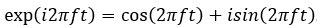.

This sort of simplification - reducing the number of symbols and terms in an expression - is an important goal in mathematics. Even for data that are not complex, the "exp" notation is more compact and elegant than "cos+sin". Many computer languages can handle complex arithmetic automatically when the quantities are complex. But this terminology can be misleading: the sine and cosine parts are equally important; just because the two parts are called "real" and "imaginary" in mathematics does not imply that the first is less practically significant that the second. For a rigorous explanation of the mathematics, see Fourier Transforms by Gary Knott.

The concept of the Fourier transform is involved in two very important instrumental methods in chemistry. In Fourier transform infrared spectroscopy (FTIR), the Fourier transform of the spectrum is measured directly by the instrument, as the interferogram formed by plotting the detector signal vs mirror displacement in a scanning Michaelson interferometer. In Fourier Transform Nuclear Magnetic Resonance spectroscopy (FTNMR), excitation of the sample by an intense, short pulse of radio frequency energy produces a free induction decay signal that is the Fourier transform of the resonance spectrum. In both cases the instrument recovers the spectrum by inverse Fourier transformation of the measured (interferogram or free induction decay) signal.

The power spectrum or frequency spectrum is a simple way of showing the total amplitude at each of these frequencies; it is calculated as the square root of the sum of the squares of the coefficients of the sine and cosine components. The power spectrum retains the frequency information but discards the phase information, so that the power spectrum of a sine wave would be the same as that of a cosine wave of the same frequency, even though the complete Fourier transforms of sine and cosine waves are different in phase. In situations where the phase components of a signal are the major source of noise (e.g. random shifts in the horizontal x-axis position of the signal), it can be advantageous to base measurement on the power spectrum, which discards the phase information, by ensemble averaging the power spectra of repeated signals: this is demonstrated by the Matlab/Octave scripts EnsembleAverageFFT.m and EnsembleAverageFFTGaussian.m.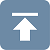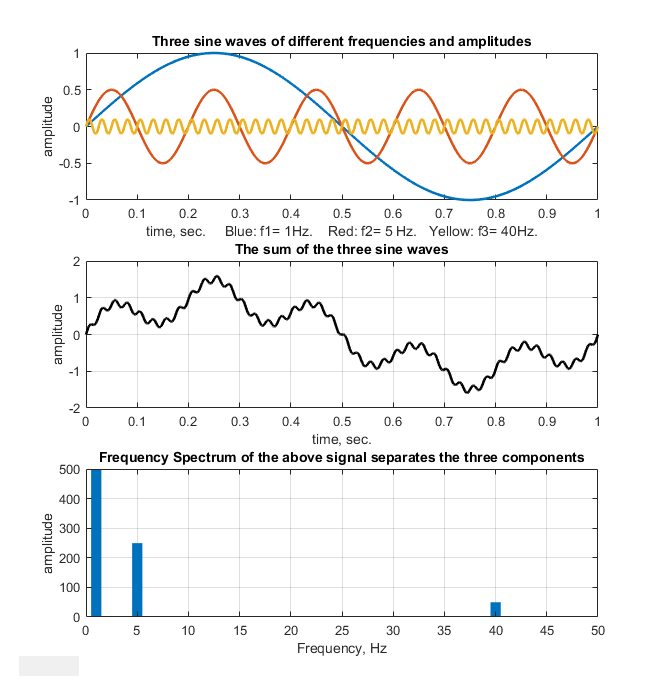A time-series signal with n points gives a power spectrum with only (n/2)+1 points. The first point is the zero-frequency (constant) component, corresponding to the DC (direct current) component of the signal. The second point corresponds to a frequency of 1/nΔx (whose period is exactly equal to the time duration of the data), the next point to 2/nΔx, the next point to 3/nΔx, etc., where Δx is the interval between adjacent x-axis values and n is the total number of points. The last (highest frequency) point in the power spectrum (n/2)/nΔx=1/2Δx, which is one-half the sampling rate. This is illustrated in the figure on the right, which shows a one-second, 1000-point signal that has only three non-zero Fourier components (top panel), all of which are clearly distinguishable in the signal itself (middle panel). The frequencies are labeled and they all show up at the expected places, and with the expected amplitudes in the Fourier spectrum, which I have drawn here as a bar graph (botom panel). You can even count the cycles of the sine components to confirm their frequencies.

The highest frequency that can be represented in a discretely-sampled waveform is one-half the sampling frequency, which is called the Nyquist frequency; frequencies above the Nyquist frequency are "folded back" to lower frequencies, severely distorting the signal. The frequency resolution, that is, the difference between the frequencies of adjacent points in the calculated frequency spectrum, is simply the reciprocal of the time duration of the signal.

A pure sine or cosine wave that has an exactly integral number of cycles within the recorded signal will have a single non-zero Fourier component corresponding to its frequency. Conversely, a signal consisting of zeros everywhere except at a single point, called a delta function, has equal Fourier components at all frequencies. Random noise also has a power spectrum that is spread out over a wide frequency range, but shaped according to its noise color, with pink noise having more power at low frequencies, blue noise having more power at high frequencies, and white noise having roughly the same power at all frequencies.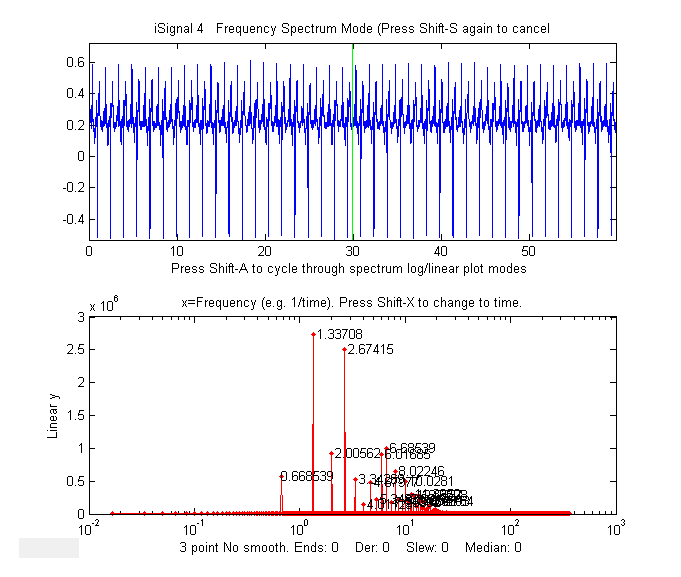For periodic waveforms that repeat over time, a single period is the smallest repeating unit of the signal, and the reciprocal of that period is called the fundamental frequency. Non-sinusoidal periodic waveforms exhibit a series of frequency components that are multiples of the fundamental frequency; there are called "harmonics". A familiar example is the electrical recording of a heartbeat, call an electrocardiograph (ECG), which consists of a highly repeatable series of waveforms, as in the real data example on the left, which shows a fundamental frequency of 0.6685 Hz with multiple harmonics at frequencies that are x2, x3, x4..., etc, times the fundamental frequency. The waveform is shown in blue in the top panel and its frequency spectrum is shown in red in the bottom panel. The fundamental and the harmonics are sharp peaks, labeled with their frequencies. The spectrum is qualitatively similar to what is obtained for perfectly regular identical peaks.

Recorded vocal sounds, especially vowels, also have a periodic waveform with harmonics. (The sharpness of the peaks in these spectra shows that the amplitude and the frequency are very constant over the recording interval in this example. Changes in amplitude or frequency over the recording interval will produce clusters or bands of Fourier components rather than sharp peaks, as in this example).

Another familiar example of periodic change is the seasonal variation in temperature, for example the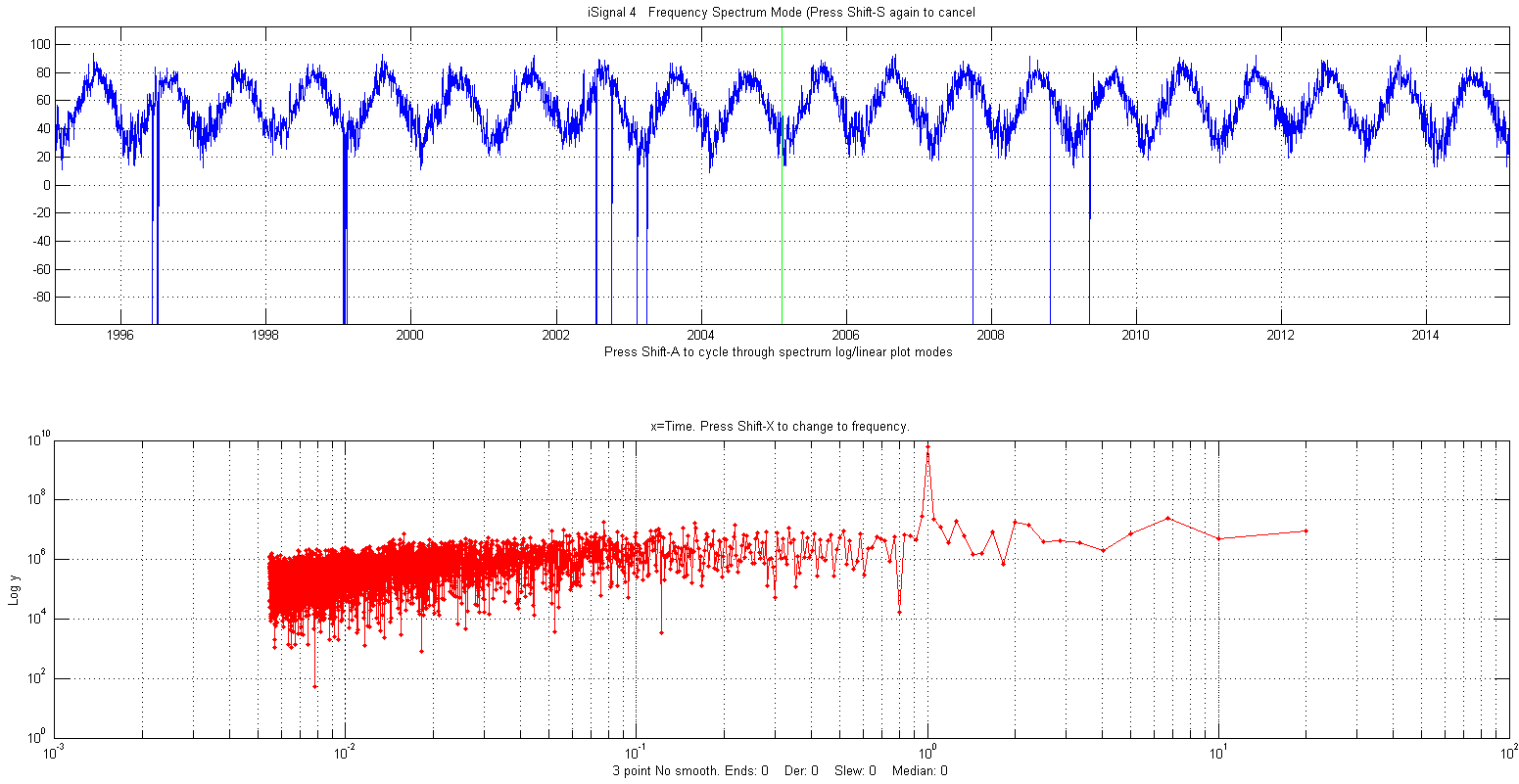average daily temperature measured in New York City between 1995 and 2015, shown in the figure on the right. (The negative spikes are missing data points - power outages?) In this example the spectrum in the lower panel is plotted with time (the reciprocal of frequency) on the x-axis (called a periodogram) which, despite the considerable random noise due to local weather variations and missing data, shows the expected peak at exactly 1 year; that peak is sharp because the periodicity is extremely (in fact, astronomically) precise. In contrast, the random noise is not periodic and is spread out roughly equally over the entire periodogram.The figure on the right is a simulation that shows how hard it is to see a periodic component in the presence of random noise, and yet how easy it is to pick it out in the frequency spectrum. In this example, the signal (top panel) contains an equal mixture of random white noise and a single sine wave; the sine wave is almost completely obscured by the random noise. The frequen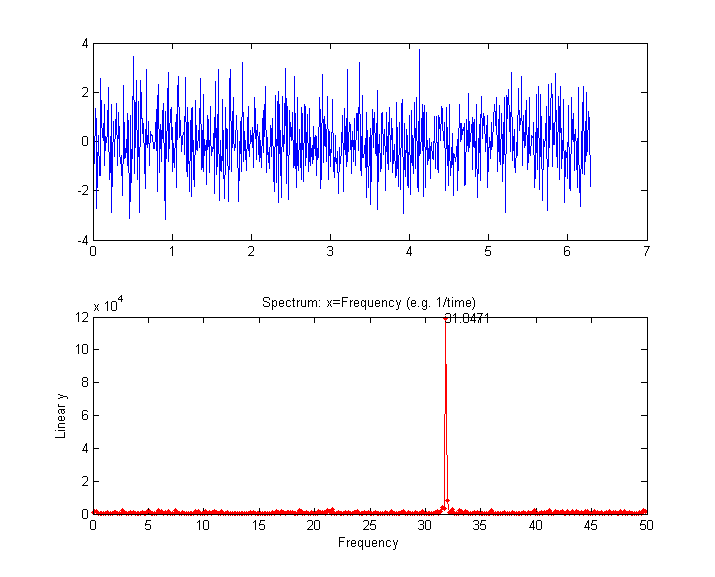cy spectrum (created using the downloadable Matlab/Octave function "PlotFrequencySpectrum") is shown in the bottom panel. The frequency spectrum of the white noise is spread out evenly over the entire spectrum, whereas the sine wave is concentrated into a single spectral element, where it stands out clearly. Here is the Matlab/Octave code that generated that figure; you can Copy and Paste it into Matlab/Octave:

x=[0:.01:2*pi]';
y=sin(200*x)+randn(size(x));
subplot(2,1,1);
plot(x,y);
subplot(2,1,2);
PowerSpectrum=PlotFrequencySpectrum(x,y,1,0,1);

A common practical application is the use of the power spectrum as a diagnostic tool to distinguish between signal and noise components. An example is the AC power-line pickup depicted in the figure below, which has a fundamental frequency of 60 Hz in the USA (why that frequency?)  or 50 Hz in some countries. Again, the sharpness of the peaks in the spectrum shows that the amplitude and the frequency are very constant; power companies take pains to keep the frequency of the AC very constant to avoid problems between different sections of the power grid. Other examples of signals and their frequency spectra are shown below).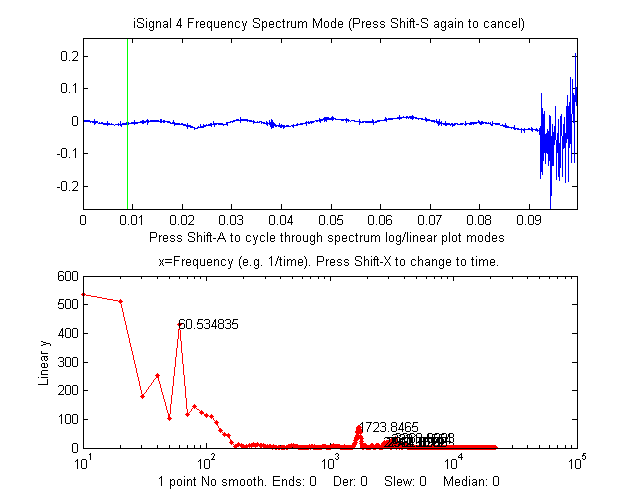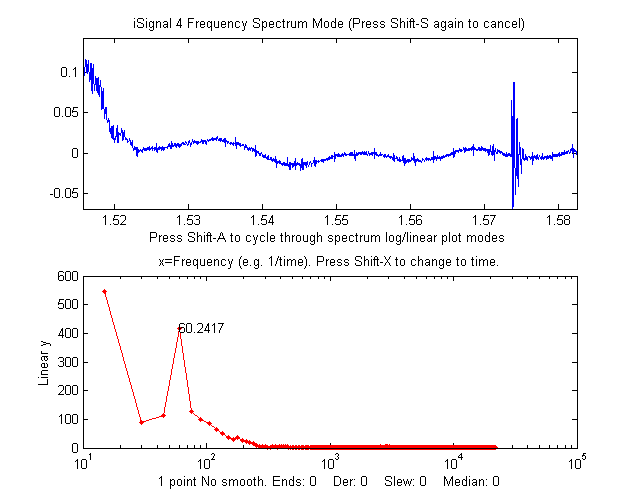Data from an audio recording, zoomed in to the period immediately before (left) and after (right) the actual sound, shows a regular sinusoidal oscillation (x = time in seconds). In the lower panel, the power spectrum of each signal (x = frequency in Hz) shows a strong sharp peak very near 60 Hz, suggesting that the oscillation is caused by stray pick-up from the 60 Hz power line in the USA (it would be 50 Hz had the recording been made in Europe). Improved shielding and grounding of the equipment might reduce this interference. The "before" spectrum, on the left, has a frequency resolution of only 10 Hz (the reciprocal of the recording time of about 0.1 seconds) and it includes only about 6 cycles of the 60 Hz frequency (which is why that peak in the spectrum is the 6th point); to achieve a better resolution you would have had to have begun the recording earlier, to achieve a longer recording. The "after" spectrum, on the right, has an even shorter recording time and thus a poorer frequency resolution.Peak-type signals have power spectra that are concentrated in a range of low frequencies, whereas random noise is often spread out over a much wider frequency range. This is why smoothing (low-pass filtering) can make a noisy signal look nicer, but also why smoothing does not usually help with quantitative measurement, because most of the peak information is found at low frequencies, where low-frequency noise remains unchanged by smoothing (See CurveFittingC.html#Smoothing).

The figures above show a classic example of harmonic analysis; it shows the annual variation in the number of observed sunspots, which have been recorded since the year 1700! In this case the time axis is in years (top window). A plot of the power spectrum (bottom window, left) shows a strong peak at 0.09 cycles/year and the periodogram (right) shows a peak at the well-known 11-year cycle, plus some evidence of a weaker cycle at around a 100-year period. (You can download this data set or the latest yearly sunspot data from NOAA. These frequency spectra are plotted using the downloadable Matlab function iSignal. In this case, the peaks in the spectrum are not sharp single peaks, but rather form a cluster of Fourier components, because the amplitude and the frequency are not constant over the nearly 300 year interval of the data, as is obvious by inspecting the data in the time domain.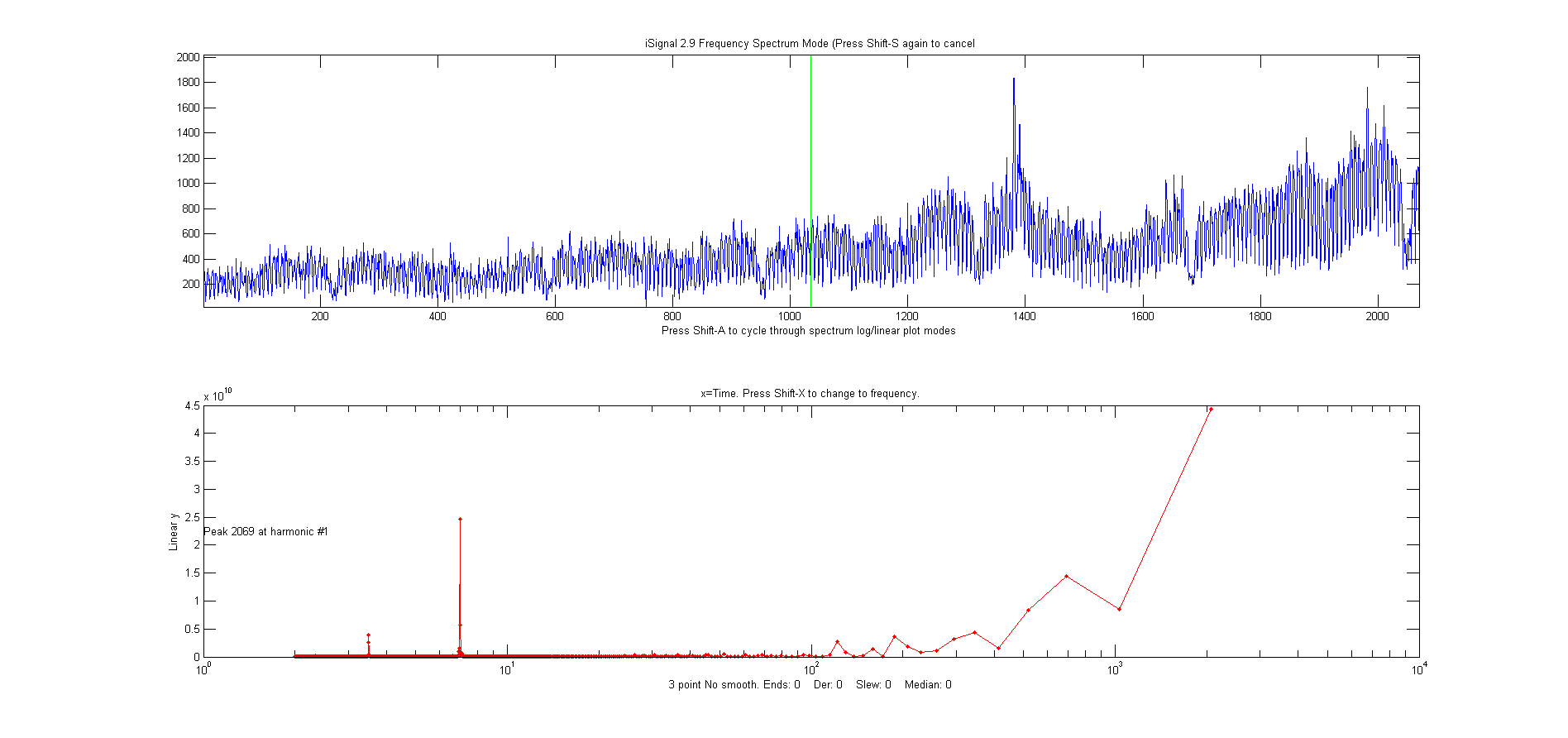An example of a time series with complex multiple periodicity is the world-wide daily page views (x=days, y=page views) for this web site over a 2070-day period (about 5.5 years). In the periodogram plot (shown on the left) you can clearly see at sharp peaks at 7 and 3.5 days, corresponding to the first and second harmonics of the expected workday/weekend cycle, and smaller peaks at 365 days (corresponding to a sharp dip each year during the winter holidays) and at 182 days (roughly a half-year), probably caused by increased use in the two-per-year semester cycle at universities. (The large values at the longest times are caused by the gradual increase in use over the entire data record, which can be thought of as a very low-frequency component whose period is much longer that the entire data record).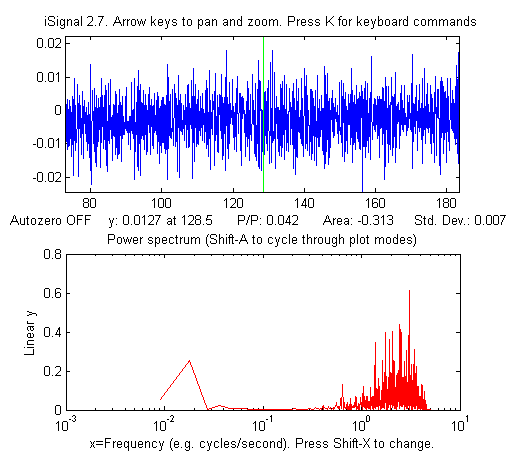In the example shown on the right, the signal (in the top window) contains no visually evident periodic components; it seems to be just random noise. However, the frequency spectrum (in the bottom window) shows that there is much more to this signal than meets the eye. There are two major frequency components: one at low frequencies around 0.02 and the other at high frequencies between 0.5 and 5. (If the x-axis units of the signal plot had been seconds, the units of the frequency spectrum plot would be Hz; note that the x-axis is logarithmic). In this particular case, the lower frequency component is in fact the signal, and the higher frequency component is residual blue noise left over from previous signal processing operations. The two components are fortunately well separated on the frequency axis, suggesting that low-pass filtering (i.e smoothing) will able to remove the noise without distorting the signal.

In the examples shown above, the signals are time-series signals with time as the independent variable. More generally, it is also possible to compute the Fourier transform and power spectrum of any signal, such as an optical spectrum, where the independent variable might be wavelength or wavenumber, or an electrochemical signal, where the independent variable might be volts, or a spacial signal, where the independent variable might be in length units. In such cases the units of the x-axis of the power spectrum are simply the reciprocal of the units of the x-axis of the original signal (e.g. nm-1 for a signal whose x-axis is in nm).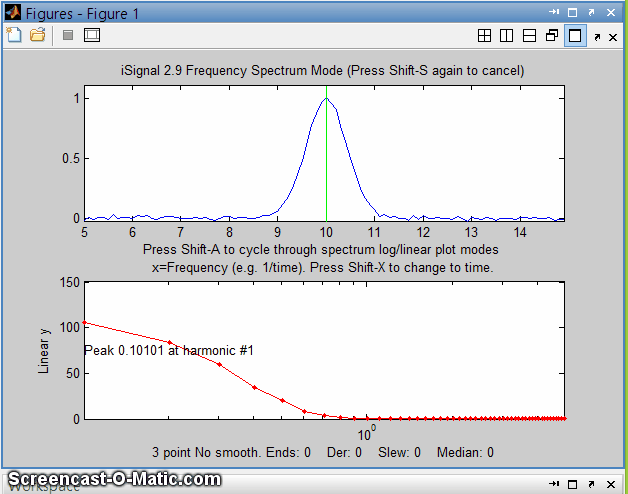Analysis of  the frequency spectra of signals provides another way to understand signal-to-noise ratio, filtering, smoothing, and differentiation. Smoothing is a form of low-pass filtering, reducing the high-frequency components of a signal. If a signal consists of smooth features, such as Gaussian peaks, then its spectrum will be concentrated mainly at low frequencies. The wider the width of the peak, the more concentrated the frequency spectrum will be at low frequencies (see animated picture on the right). If that signal is contaminated with white noise (spread out evenly over all frequencies), then smoothing will make the signal look better, because it reduces the high-frequency components of the noise. However, the low-frequency noise will remain in the signal after smoothing, where it will continue to interfere with the measurement of signal parameters such as peak heights, positions, widths, and areas. This can be demonstrated by least-squares measurement.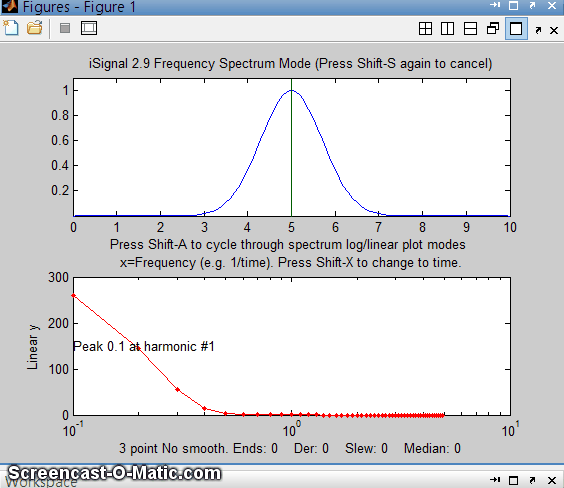Conversely, differentiation is a form of high-pass filtering, reducing the low frequency components of a signal and emphasizing any high-frequency components present in the signal. A simple computer-generated Gaussian peak (shown by the animation on the left) has most of its power concentrated in just a few low frequencies, but as successive orders of differentiation are applied, the waveform of the derivative swings from positive to negative like a sine wave, and its frequency spectrum shifts progressively to higher frequencies, as shown in the animation on the left. This behavior is typical of any signal with smooth peaks. So the optimum range for signal information of a differentiated signal is restricted to a relatively narrow range, with little useful information above and below that range.

The fact that white noise is spread out in the frequency domain roughly equally over all frequencies has a subtle advantage over other noise colors when the signal and the noise can not be cleanly separated in the time domain; you can more easily estimate the intensity of the noise by observing it in frequency regions where the signal does not interfere, since most signals do not occupy the entire spectrum frequency range. This idea will be put to use later as a method for estimating the errors of measurements based on least-squares curve fitting of noisy data.

The presence of multiple peaks results in a more complex frequency spectrum with multiple peaks (script).

The measurement of overlapping peaks is easier and more accurate if the peaks are narrow (script). The method of artificial (i.e. computational) peak sharpening by the even-derivative method, introduced in the previous section, emphasizes the high frequency components by adding a portion of the second and fourth derivatives to the original signal. You can see this clearly in the script PeakSharpeningFrequencySpectrum.m, which shows the frequency spectrum of the original and sharpened version of a signal consisting of several peaks (graphic).

SineToDelta.m. A demonstration animation showing the waveform and the power spectrum of a rectangular pulsed sine wave of variable duration (whose power spectrum is a "sinc" function) changing continuously from a pure sine wave at one extreme (where its power spectrum is a delta function) to a single-point pulse at the other extreme (where its power spectrum is a flat line). GaussianSineToDelta.m is similar, except that it shows a Gaussian pulsed sine wave, whose power spectrum is a Gaussian function, but which is the same at the two extremes of pulse duration (animated graphic).

Real experimental signals are often contaminated with drift and baseline shift, which are essentially low-frequency effects, and random noise, which is usually spread out over all frequencies. For these reasons, differentiation is always used in conjunction with smoothing.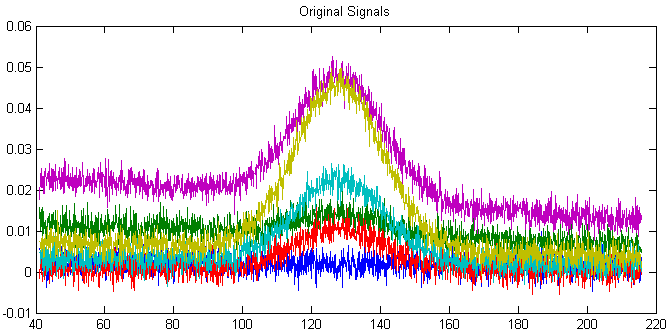Working together, smoothing and differentiation act as a kind of frequency-selective bandpass filter that optimally passes the band of frequencies containing the differentiated signal information but reduces both the lower-frequency effects, such as slowly-changing drift and background, as well as the high-frequency noise. An example of this can be seen in the DerivativeDemo.m described in a previous section. In the set of six original signals, shown on the right, the random noise occurs mostly in a high frequency range, with many cycles over the x-axis range, and the baseline shift occurs mostly in a much lower-frequency phenomenon, with only a small fraction of one cycle occurring over that range. In contrast, the peak of interest, in the center of the x-range, occupies an intermediate frequency range, with a few cycles over that range. Therefore we could predict that a quantitative measure based on differentiation and smoothing might work well, as was shown previously.

Smoothing and differentiation change the amplitudes of the various frequency components of signals, but they do not change of shift the frequencies themselves. An experiment described later illustrates this idea using a brief recording of speech. Interestingly, different degrees of smoothing and differentiation will change timbre of the voice but has little effect on the intelligibility; the sequence of pitches is not shifted in pitch or time but merely changed in amplitude by smoothing and differentiation. Because of this, recorded speech can survive digitization, transmission over long distances, and playback via tiny speakers and headphones without significant loss of intelligibility. Music, on the other hand, suffers greater loss under such circumstances, as you can tell by listening to typically terrible telephone "hold" music.

### Software detailsIn a spreadsheet or computer language, a sine wave can be described by the 'sin' function y=sin(2*pi*f*x+p) or y=sin(2*pi*(1/t)*x+p), where pi is 3.14159...., f is frequency of the waveform, t is the period of the waveform, p is the phase, and x is the independent variable (usually time).

There are several Web sites that can compute Fourier transforms interactively (e.g. WolframAlpha).

Microsoft Excel has a add-in function that makes it possible to perform Fourier transforms relatively easily: (Click Tools > Add-Ins... > Analysis Toolpak > Fourier Analysis).  See "Excel and Fourier" for details. See http://www.bowdoin.edu/~rdelevie/excellaneous/ for an extensive and excellent collection of add-in functions and macros for Excel, courtesy of Dr. Robert deLevie of Bowdoin College.

There are a number of dedicated FFT spectral analysis programs, including ScopeDSP (https://iowegian.com/scopedsp/) and Audacity (http://sourceforge.net/projects/audacity/).

SPECTRUM, the freeware signal-processing application for Macintosh OS8, includes a power spectrum function, as well as forward and reverse Fourier transformation.Matlab and Octave have built-in functions for computing the Fourier transform (fft and ifft). These function express their results as complex numbers. For example, if we compute the Fourier transform of a simple 3-element vector, we get 3-element result of complex numbers:

y=[0 1 0];
fft(y)

ans =
1.0000    -0.5000-0.8660i    -0.5000+0.8660i
where the "i" indicates the "imaginary" part. The first element of the fft is just the sum of elements in y. The inverse fft , ifft([1.0000    -0.5000-0.8660i    -0.5000+0.8660i]), returns the original vector [0 1 0]. For another example, the fft of  [0 1 0 1] is [2     0    -2     0]. In general, the fft of an n-element vector of real numbers returns an n-element vector of real or complex numbers, but only the first n/2+1 elements are unique; the remainder are a mirror image of the first. Operations on individual elements of the fft, such as in Fourier filtering, must take this structure into account.

The frequency spectrum of a signal vector "s" can be computed as real(sqrt(fft(s) .* conj(fft(s)))). Here's a simple example where we know the answer in advance, at least qualitatively: an 8-element vector of integers that trace out a single cycle of a sine wave:

s=[0 7 10 7 0 -7 -10 -7];
plot(s);
real(sqrt(fft(s) .* conj(fft(s))))

The frequency spectrum in this case is [0 39.9 0 0.201 0 0.201 0 39.9]. Again, the first element is the average (which is zero) and elements 2 through 4 are the mirror image of the last 4. The unique elements are the first four, which are the amplitudes of the sine wave components whose frequencies are 0, 1, 2, 3 times the frequency of a sine wave that would just fit a single cycle in the period of the signal. In this case it the second element (39.8) that is the largest by far, which is just what we would expect for a signal that approximates a single cycle of a sine (rather than a cosine) wave. Had the signal been two cycles of a sine wave, s=[0 10 0 -10 0 10 0 -10], the third element would have been the strongest (try it). The highest frequency that can be represented by an 8-element vector is one that has a period equal to 2 elements. It takes a minimum of 4 points to represent one cycle, e.g. [0  +1  0  -1].

Here's a Matlab script that creates and plots a sine wave and then uses the fft function to calculate and plot the power spectrum. Try different frequencies (third line). Watch what happens when the frequency approaches 50. Hint: the Nyquist frequency is 1/(2*Deltat) = 1/0.02=50. Also, see what happens when you change Deltat (first line).

Deltat=.01; % Sampling interval (1/sample rate)
t=[0:Deltat:2*pi]'; % time axis

frequency=25; % Frequency
y=sin(2*pi*frequency*t); % Create a sine wave of that frequency
subplot(2,1,1); % Plot and label it in upper half of figure window
plot(t,y);
title('Signal')
xlabel('time, t')
fy=fft(y); % Compute Fourier transform
sy=fy .* conj(fy); % Multiply by complex conjugate
plotrange=1:length(fy)/2; % Define plotrange as half length of y
ps=real(sy(plotrange)); % ps = power spectrum
f=(plotrange-1)./range(t); % Create frequency axis (reciprocal of t)
subplot(2,1,2); % Plot and label it in lower half of figure window
plot(f,ps)
title('Power spectrum')
xlabel('frequency, f')

More simply, the downloadable function FrequencySpectrum.m (syntax fs=FrequencySpectrum(x,y)) returns real part of the Fourier power spectrum of x,y as a matrix.

x=0:.01:2*pi;
f=25; % f=frequency
y=sin(2*pi*f*x)+randn(size(x))
fs=FrequencySpectrum(x,y);
plot(fs)

The plot of the frequency spectrum fs shows a single strong peak at f=25. The frequency of the strongest peak in fs can be calculated with fs(val2ind(fs(:,2),max(fs(:,2))),1)

PlotFrequencySpectrum.m can plot frequency spectra and periodograms on linear or log coordinates in one step. Type "help PlotFrequencySpectrum".

For some other examples of using FFT example, see these examples. A "Slow Fourier Transform" function has also been published; it is 8000 times slower with a 10,000-point data vector, as can be shown by this bit of code: y=cos(.1:.01:100); tic; fft(y); ffttime=toc; tic; sft(y); sfttime=toc; TimeRatio=sfttime/ffttime

In Python, you first must execute "from scipy import fft", then the syntax is fft.fft(y) or fft.ifft(y) for the forward and inverse fft of y, respectively.

Time-segmented Fourier power spectrum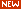PlotSegFreqSpect.m, PSM=PlotSegFreqSpect(x, y, NumSegments, MaxHarmonic, logmode), version 1.3, creates and displays a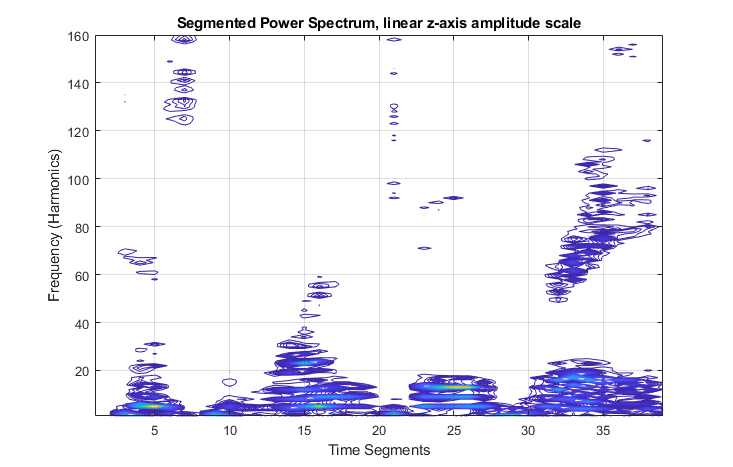time-segmented Fourier power spectrum, also known as a "Short-Time Fourier transform (STFT)". It breaks y into 'NumSegments' equal-length segments, computes the power spectrum of each segment, and plots the result of the first 'MaxHarmonic' Fourier components as a contour plot. If the number of segments and of data points is such that the last segment is incomplete, it is discarded. The function returns the power spectrum matrix (time-frequency-amplitude) as a matrix of size (NumSegments x MaxHarmonic). If logmode=1, it computes and plots the base10 logarithm of the amplitudes, and displays the matrix as a contour plot, with yellow representing higher amplitudes and green and blue lower amplitudes. Typing "mesh(PSM)"  shows the 3-D mesh plot of the power spectrum matrix, which can be rotated by dragging the pointer. Examples in the help file include the spectrum of a passing automobile horn and of a brief sample of human speech shown on the left.

Example 6 in the help file of PlotSegFreqSpect is shown in the figures below; it shows a very weak signal consisting of the sum of three components shown in the top left: (1) two Gaussian peaks (blue), which represent the desirable signal that I want to measure, (2) a  strong variable-frequency sine wave (red), and (3) random noise (green). The sine wave and the noise are both much stronger than the two Gaussian peaks. I'll call this the "buried peaks" signal, and I'll use it again later. We have to pretend that we don't know any of that and look only at the raw signal. In fact, the Gaussian peaks are so weak that they invisible in the raw signal (top right), but when the segmented frequency spectrum is computed (bottom center), two yellow blobs show up at x= 2 and 4, y=1, along the bottom of the segmented spectrum (right), clearly distinct from the much stronger diagonal stripe of yellow and white from the swept sine wave. (In these contour plots, yellow represents a higher amplitude than green or blue). Once you see those blobs, you can constrain the range of further observation there, and the peaks could be verified by smoothing or could even be measured quantitatively by curve-fitting the raw data (not the smoothed data, to avoid the distortion caused by smoothing). In fact, the curve fitting results give fairly good values (plus or minus 10% or better) for the peak positions, heights, and widths, despite the invisibility of the peaks in the raw data. But you have to be able see the peaks first, and estimate their number and shape and location, before you could proceed with curve fitting.

### iSignal: Interactive Signal Processing tooliSignal is a downloadable interactive multipurpose signal processing Matlab function that includes a Frequency Spectrum mode, toggled on and off by the Shift-S key; it computes frequency spectrum of the segment of the signal displayed in the upper window and displays it in the lower window (in red). You can use the pan and zoom keys to adjust the region of the signal to be viewed or press Ctrl-A to select the entire signal. Press Shift-S again to return to the normal mode.

In the frequency spectrum mode, you can press Shift-A to cycle through four plot modes (linear, semilog X, semilog Y, or log-log). Because of the wide range of amplitudes and frequencies exhibited by some signals, the log plot modes often results in a clearer graph that the linear modes. You can also press Shift-X to toggle between frequency on the x axis and time on the x-axis. The example on the right shows the frequency mode. Press Shift-Z to display the frequencies of the main harmonics on each peak (as in the ECG example at the top of this page).

### Frequency visualization.

What happens if the frequency content changes with time? Consider, for example, the signal shown in the following figure. The signal (SineBursts.mat) consists of three intermittent bursts of sinewaves of three different frequencies, with zeros between the bursts.

Here the signal is shown in the upper panel in the iSignal.m function by typing

>> isignal(x,y);

at the Matlab command prompt.

By pressing Shift-S, its frequency spectrum is displayed in the lower panel, which shows three discreet frequency components. But which one is which? The normal Fourier transform by itself offers no clue, but iSignal allows you to pan and zoom across the signal, using the cursor arrow keys, so you would be able to isolate each.

The downloadable function PlotSegFreqSpec.m, described in the previous section (figures below) is another way to display this signal in a single graphic that clearly displays the time and frequency variation of the signal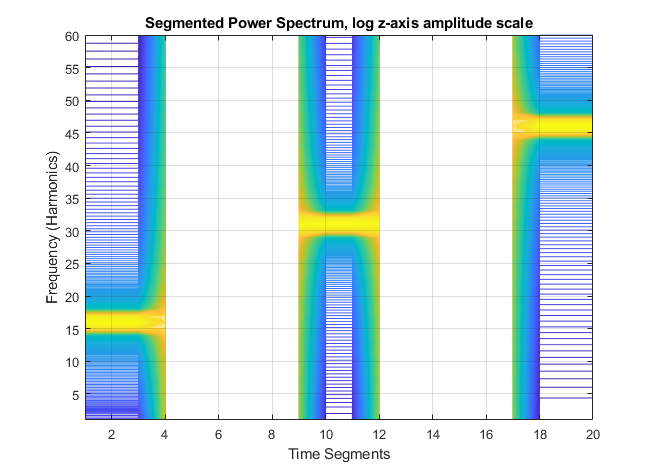You can do a similar visualization with Matlab's inbuilt "Short Time Fourier Transform" function stft.m (below), which displays both positive and negative frequencies.

>> stft(y)

### Signal enhancement

An important feature of iSignal is that all signal processing functions remain active in the frequency spectrum mode (smooth, derivative, etc.), so you can observe the effect of these functions on the frequency spectrum of the signal immediately. The figure below shows the effect of increasing the smooth width on the 2nd derivative of a signal containing three weak noisy peaks. Without smoothing, the signal seems to be all random noise; with enough smoothing, the three weak peaks are clearly visible (in derivative form) and measurable.

###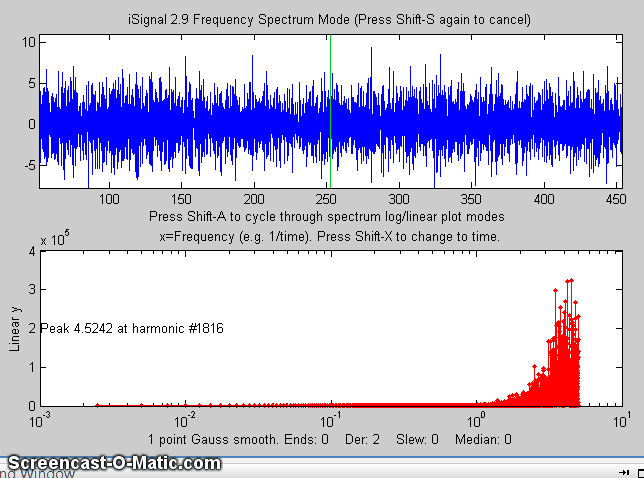In the next example below, the signal is a pair of Lorentzian peaks which are initially completely obscured by a strongly periodic noise source that causes a rough peak in the frequency spectrum (bottom panel). As the smooth width increases, the actual signal gradually emerges from the noise, but if the smooth width is too great, the peaks are broadened and shortened.

Details and instructions for iSignal are here. View the code here or download the ZIP file with sample data for testing.

The script "iSignalDeltaTest" demonstrates the frequency response of the smoothing and differentiation functions of iSignal by a applying them to a delta function. Change the smooth type, smooth width, and derivative order and see how the power spectrum changes.

### Demonstration that the Fourier frequency spectrum of a Gaussian is also a Gaussian:

x=-100:.2:100;
width=2;y=gaussian(x,0,
width);
isignal([x;y],0,400,0,3,0,0,0,10,1000,0,0
,1);

Click on the figure window, press Shift-T to transfer the frequency spectrum to the top panel, then press Shift-F, press Enter three times, and click on the peak in the upper window. The program computes a least-squares fit of a Gaussian model to the frequency spectrum now in the top panel. The fit is essentially perfect:

Least-squares fit of selected peaks to Gaussian peak model using the peakfit function:
Fitting Error = 6.4221e-007%     R2 = 1
Peak#     Position     Height      Width         Area
1   -6.2545e-009     113.31      0.31265       17.721

If you repeat this with Gaussians of different widths (e.g. width=1 or 4), you'll find that that the width of the frequency spectrum peak is inversely proportional to the width of the signal peak.

### Power Spectrum Demo for Matlab (version 2)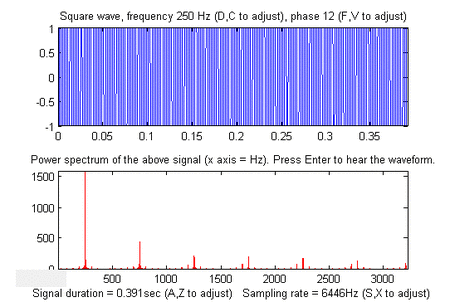Keyboard-operated version for Matlab 7.8 Slider-operated version for Matlab 6.5 iPower: Keyboard-controlled interactive power spectrumdemonstrator, useful for teaching and learning about the power spectra of different types of signals and the effect of signal duration and sampling rate. Single keystrokes allow you to select the type of signal (12 different signals included), the total duration of the signal, the sampling rate, and the global variables f1 and f2 which are used in different ways in the different signals. When the Enter key is pressed, the signal (y) is sent to the Windows WAVE audio device. Press K to see a list of all the keyboard commands. Tested in Matlab version 7.8 (R2009a). Click here to view or download.  You can also download it from the Matlab File Exchange. KEYBOARD CONTROLS:  Adjust signal duration 10% up/down.........A,Z  Adjust sampling rate 10% up/down...........S,X  Adjust first variable 10% up/down......... D,C  Adjust second variable 10% up/down........ F,V  Cycle through Linear/Log plot modes..........L  Switch X-axis scale of power spectrum........H  Print keyboard commands......................K  Play signal as sound................Enter or P                      PRE-PROGRAMMED SIGNAL TYPES *Sine wave, frequency f1 (Hz), phase f2 *Square wave, frequency f1 (Hz), phase f2 *Sawtooth wave, frequency Ff1(Hz) *Triangle wave, frequency f1 (Hz), phase f2 *Sine wave burst of frequency f1 (Hz) and length f2 sec *440 Hz carrier amplitude modulated by sine wave, frequency f1 (Hz) and amplitude f2 *440 Hz carrier frequency modulated by sine wave of frequency f1 (Hz) and amplitude f2 *Sine wave, frequency f1 (Hz), modulated with Gaussian of width f2 sec *Sine wave, frequency f1 (Hz) with non-linear transfer function f2 *Sine wave sweep from 0 to f1 (Hz) *Sine wave of frequency f1 (Hz) and amplitude f2 plus random white noise *Pink (1/f) noise *Sine wave, frequency f1 (Hz), amplitude f2 plus pink noise There is also an older slider-operated version (see left) for Matlab version 6.5. Click here to view or download.Last updated  August, 2021. This page is part of "A Pragmatic Introduction to Signal Processing", created and maintained by Prof. Tom O'Haver , Department of Chemistry and Biochemistry, The University of Maryland at College Park. Comments, suggestions and questions should be directed to Prof. O'Haver at toh@umd.edu.
Unique visits to this site since May 17, 2008: